# Criteria for Congruence of Triangles - RHS Congruence Criterion

#### definition

RHS Congruence criterion: If under a correspondence, the hypotenuse and one side of a right-angled triangle are respectively equal to the hypotenuse and one side of another right-angled triangle, then the triangles are congruent.

# RHS Congruence criterion:

If under a correspondence, the hypotenuse and one side of a right-angled triangle are respectively equal to the hypotenuse and one side of another right-angled triangle, then the triangles are congruent.

Which congruence criterion do you use in the following?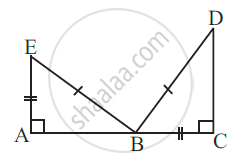Given:
EB = DB
AE = BC
∠A = ∠C = 90°
So, ∆ABE ≅ ∆CDB

RHS Congruence criterion, as in the given two right-angled triangles, one side and the hypotenuse are respectively equal.

#### Example

Given below are measurements of some parts of two triangles. Examine whether the two triangles are congruent or not, using the RHS congruence rule. In the case of congruent triangles, write the result in symbolic form:

∆ABC, ∠B = 90°, AC = 8 cm, AB = 3 cm.

∆PQR, ∠P = 90°, PR = 3 cm, QR = 8 cm.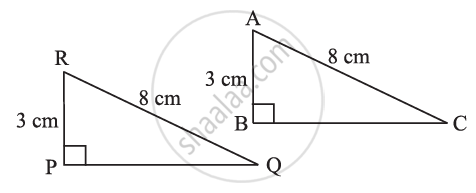Here,
∠B = ∠P = 90º,
hypotenuse, AC = hypotenuse, RQ (= 8 cm) and
side AB = side RP ( = 3 cm)

So, ∆ABC ≅ ∆RPQ.....................(By RHS Congruence rule).

#### Example

Given below are measurements of some parts of two triangles. Examine whether the two triangles are congruent or not, using the RHS congruence rule. In the case of congruent triangles, write the result in symbolic form:

∆ABC, ∠A = 90°, AC = 5 cm, BC = 9 cm.

∆PQR, ∠Q = 90°, PR = 8 cm, PQ = 5 cm.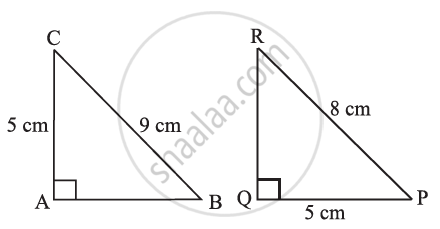Here, ∠A = ∠Q (= 90°) and side AC = side PQ ( = 5 cm).
But hypotenuse BC ≠ hypotenuse PR.
So, the triangles are not congruent.

#### Example

In Fig, DA ⊥ AB, CB ⊥ AB, and AC = BD. State the three pairs of equal parts in ∆ABC and ∆DAB. Which of the following statements is meaningful?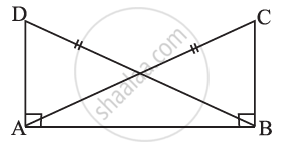(ii) ∆ABC ≅ ∆ABD.

The three pairs of equal parts are:
AC = BD...........................(Given)
AB = BA...........................(Common side)
From the above,
∆ABC ≅ ∆BAD..............(By RHS congruence rule).

So, statement (i) is true.
Statement (ii) is not meaningful, in the sense that the correspondence among the vertices is not satisfied.

#### Example

In Fig,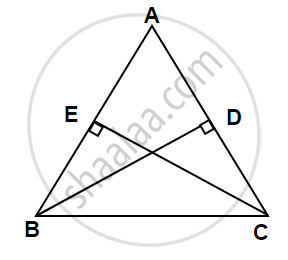BD and CE are altitudes of ∆ABC such that BD = CE.
(i) State the three pairs of equal parts in ∆CBD and ∆BCE.
(ii) Is ∆CBD ≅ ∆BCE? Why or why not?
(iii) Is ∠DCB = ∠EBC? Why or why not?

(i)
In ∆CBD and ∆BCE,
∠D = ∠E = 90°
Side BC = Side BC
Side BD = Side CE.

(ii) ∆CBD ≅ ∆BCE.........[From (i), RHS Congruence Criterion]
(iii) ∠DCB = ∠EBC.......(Corresponding Angle Congruence Test)

#### Example

ABC is an isosceles triangle with AB = AC and AD is one of its altitudes.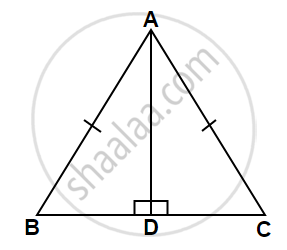(i) State the three pairs of equal parts in ∆ADB and ∆ADC.
(iii) Is ∠B = ∠C? Why or why not?
(iv) Is BD = CD? Why or why not?

∠BDA = ∠CDA.................(each 90°)
Side AB = Side AC..........(Given)

(iii) ∠B = ∠C........(Corresponding Angle Congruence test)

(iv) BD = CD........(Corresponding Side Congruence test)

If you would like to contribute notes or other learning material, please submit them using the button below.

### Shaalaa.com

RHS Congruence Criterion [00:04:20]
S
0%